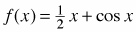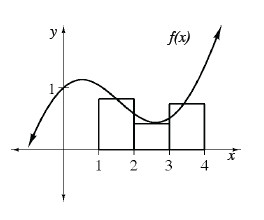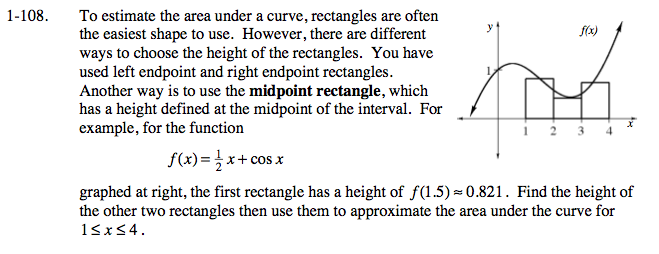### Home > CALC > Chapter 1 > Lesson 1.3.1 > Problem1-108

1-108.
1. To estimate the area under a curve, rectangles are often the easiest shape to use. However, there are different ways to choose the height of the rectangles. You have used left endpoint and right endpoint rectangles. Another way is to use the midpoint rectangle, which has a height defined at the midpoint of the interval. For example, for the functiongraphed below, the first rectangle has a height of f(1.5) ≈ 0.821. Find the height of the other two rectangles then use them to approximate the area under the curve for 1 ≤ x ≤ 4. Homework Help ✎Use your calculator to find the values of f(2.5) and f(3.5).

The base of each rectangle is 1. The aproximation for the area under the curve is A = 1(f(1.5) + f(2.5) + f(3.5)).